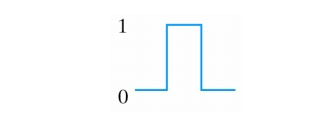Home | | Digital Electronics | Hazards in Combinational Circuits and Sequential Circuits

# Hazards in Combinational Circuits and Sequential Circuits

Hazards are unwanted switching transients that may appear at the output of a circuit because different paths exhibit different propagation delays.

HAZARDS

Hazards are unwanted switching transients that may appear at the output of a circuit because different paths exhibit different propagation delays.

Hazards occur in combinational circuits, where they may cause a temporary false-output value. When this condition occurs in asynchronous sequential circuits, it may result in a transition to a wrong stable state.

Hazards in Combinational Circuits

The following circuit demonstrates the occurrence of a hazard:Assume that all three inputs are initially equal to 1. Then consider a change of x2 from 1 to 0. The output momentarily may go to 0 if the propagation through the inverter is taken into account.

The circuit implements the Boolean function in  sum-of-products

Y = x1x2 + x2’ x3

This type of implementation may cause the output to go to 0 when it should remain a 1. This is known as a static 1-hazard:If the circuit was implemented in product-of-sums, namely:

Y = (x1 + x2’ )(x2 + x3 )

Then the output may momentarily go to 1 when it should remain 0. This is referred to as a static 0-hazard:A third type of hazard, known as dynamic hazard causes the output to change 2 or 3 time when it should be change from 1 to 0 or 0 to 1:The occurrence of the hazard can be detected by inspecting the map of the particular circuit:Y = x1x2 + x2’x3

The remedy for eliminating a hazard is to enclose the two minterms in question with another product term that overlaps both groupings:Y = x1x2 + x2’x3 + x1x3

The hazard-free circuit is:Hazards in Sequential Circuits

Consider the following asynchronous sequential circuit:If the circuit is in total state yx1x2 = 111 and input x2 changes from 1 to 0, the next total state should be 110. However, because of the hazard, output Y may go 0 momentarily.

If this false signal feeds back into gate 2 before the output of the inverter goes to 1, the output of gate 2 will remain at 0 and the circuit will switch to the incorrect total state 010.

This can be eliminated by adding an extra gate.

Essential Hazards

An essential hazard is the result of the effects of a single input variable change reaching one feedback path before another feedback path.

Essential hazards cannot be corrected by adding redundant gates as in static hazards.

They can always be eliminated in a realization by the insertion of sufficient delays in the feedback paths. Facility in doing this comes only with experience.

Study Material, Lecturing Notes, Assignment, Reference, Wiki description explanation, brief detail
Digital Electronics : Synchronous and Asynchronous Sequential Circuits : Hazards in Combinational Circuits and Sequential Circuits |